## A car is moving at 12 m per 2nd and has a mass of 600 kg what is the kinetic energy of the car

Question

A car is moving at 12 m per 2nd and has a mass of 600 kg what is the kinetic energy of the car

in progress 0
4 months 2021-09-03T18:30:00+00:00 1 Answers 46 views 0

## Answers ( )

43,200 Joules

Explanation:

Kinetic energy can be found using the following formula: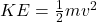where m is the mass in kilograms and v is the velocity in m/s.

We know the mass is 600 kilograms, and the car is moving at 12 meters per second. Therefore the mass is 600 kilograms and the velocity is 12 m/s.

m=600

v=12

Substitute these values into the formula.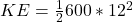First, evaluate the exponent: 12^2. 12^2 is the same as 12*12, which is equal to 144.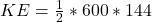Multiply 600 and 144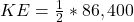Multiply 1/2 and 86,400, or divide 86,400 by 2.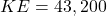Add appropriate units. Kinetic energy uses Joules, or J for units.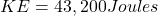The kinetic energy of the car is 43,200 Joules.